# Co Ordinate Geometry Solution of TS & AP Board Class 9 Mathematics

###### Exercise 5.1

Question 1.

In a locality, there is a main road along North-South direction. The map is given below. With the help of the picture answer the following questions.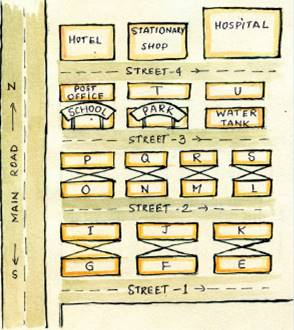(i) What is the 3rd object on the left side in street no. 3?

(ii) Find the name of the 2nd house which is in right side of street 2.

(iii) Locate the position of Mr. K’s house.

(iv) How do you describe the position of the post office?

(v) How do you describe the location of the hospital?

(i) From the given figure, we can see that the 3rd object on the left side in street no. 3 is “Water Tank”.

(ii) From the given figure, the name of the 2nd house which is in right side of street 2 is “Mr. J’s” house.

(iii) From the figure given, we see that, Mr. K’s house is the 3rd house on the right of street 2 going while towards east direction.

(iv) From the given figure, we see that the 1st building on the right side of the street 4 while going towards east is the post office.

(v) From the given figure, we see that the 3rd building on the left side of street 4 while going in the easy direction is the hospital.

###### Exercise 5.2

Question 1.

Write the quadrant in which the following points lie?

i) (-2, 3)

ii) (5, -3)

iii) (4, 2)

iv) (-7, -6)

v) (0, 8)

vi) (3, 0)

vii) (-4, 0)

viii) (0, -6)

From the figure given below, we can tell the quadrants of the given points.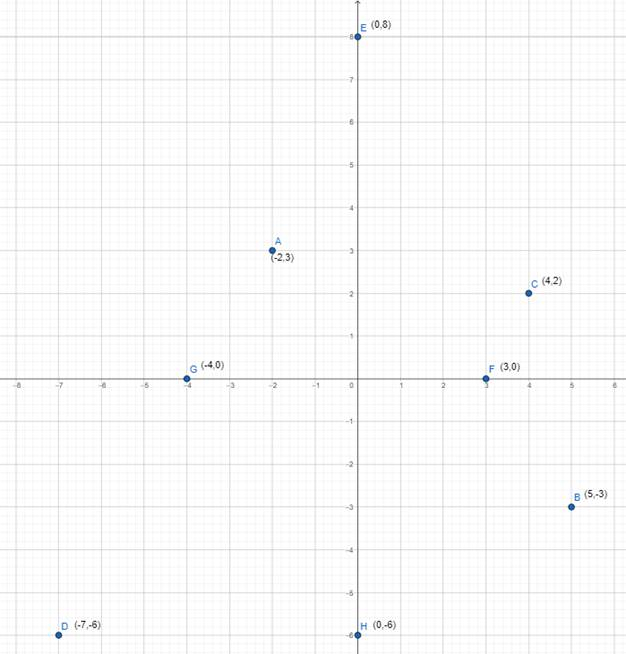(i) The point A(-2,3) lies in the II quadrant.

(ii)The point B(5,-3) lies in the IV quadrant.

(iii) The point C(4,2) lies in the I quadrant.

(iv) The point D(-7,-6) lies in the III quadrant.

(v) The point E(0,8) lies on the Y-axis.

(vi) The point F(3,0) lies on the X-axis.

(vii) The point G(-4,0) lies on the X-axis.

(viii) The point H(0,-6) lies on the Y-axis.

Question 2.

Write the abscissae and ordinates of the following points.

i) (4, -8)

ii) (-5, 3)

iii) (0, 0)

iv) (5, 0)

v) (0, -8)

Note : Plural of abscissa is abscissae.

As we know, the abscissa of a point is its x co-odinate and ordinate of a point is its y co-ordinate {if a point≡(x,y)}.

So,

(i) For the point (4,-8) :

abscissa : 4

ordinate : -8

(ii) For the point (-5,3) :

abscissa : -5

ordinate : 3

(iii) For the point (0,0) :

abscissa : 0

ordinate : 0

(iv) For the point (5,0) :

abscissa : 5

ordinate : 0

(v) For the point (0,-8) :

abscissa : 0

ordinate : -8

Question 3.

Which of the following points lie on the axes? Also name the axis.

i) (-5, -8)

ii) (0, 13)

iii) (4, -2)

iv) (-2, 0)

v) (0, -8)

vi) (7, 0)

vii) (0, 0)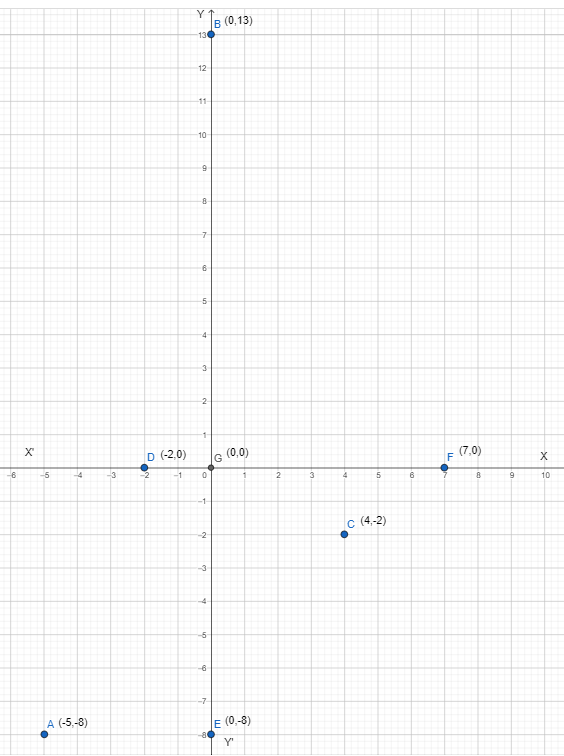From the figure above, The points which lie on axes are :

(ii) (0, 13) which lies on the Y-axis

(iv) (-2, 0) which lies on the X-axis

(v) (0, -8) which lies on the Y-axis

(vi) (7, 0) which lies on the X-axis

(vii)(0, 0) which lies on the on both the axis.

Question 4.

Write the following based on the graph.

i) The ordinate of L

ii) The ordinate of Q

iii) The point denoted by (-2, -2)

iv) The point denoted by (5, 4)

v) The abscissa of N

vi) The abscissa of M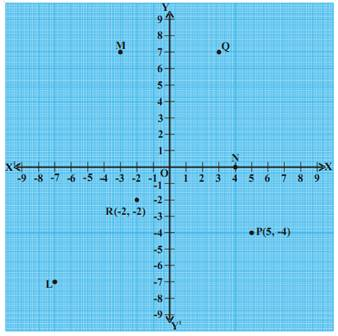From the above figure we can say that,

(i) The ordinate of point L is -7.

(ii) The ordinate of point Q is 7.

(iii) The point denoted by (-2, -2) is R.

(iv) The point denoted by (5, 4) is P.

(v) The abscissa of point N is 4.

(vi) The abscissa of point M is -3.

Question 5.

State True or False and write correct statement.

i. In the Cartesian plane the horizontal line is called Y - axis.

ii. In the Cartesian plane, the vertical line is called Y - axis.

iii. The point which lies on both the axes is called origin.

iv. The point (2, -3) lies in the third quadrant.

v. (-5, -8) lies in the fourth quadrant.

vi. The point (-x, -y) lies in the first quadrant where x <0, y < 0.

(i)The statement given is “False”.

⇒ CORRECT STATEMENT : In the Cartesian plane the horizontal line is called X - axis.

(ii) The statement given is “True”

(iii) The statement given is “True”

(iv) The statement given is “False”.

⇒ CORRECT STATEMENT : The point (2, -3) lies in the fourth quadrant.

(v) The statement given is “False”.

⇒ CORRECT STATEMENT : The point (-5, -8) lies in the second quadrant.

(vi) The statement given is “True”.

Question 6.

Plot the following ordered pairs on a graph sheet. What do you observe?

(1, 0), (3, 0), (-2, 0 ), (-5, 0), (0, 0), (5, 0), (-6, 0)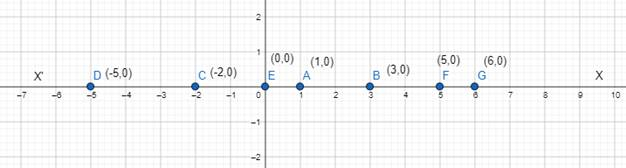The above all points lie on the X-axis.

Question 7.

Plot the following ordered pairs on a graph sheet. What do you observe?

(0, 1), (0, 3), (0, -2), (0, -5), (0, 0), (0, 5), (0, -6)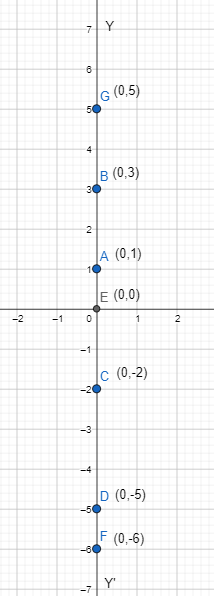The above all points lie on the Y-axis.

We also saw that a point common to both the axes was (0,0) which is the origin.

###### Exercise 5.3

Question 1.

Plot the following points in the Cartesian plane whose x, y co-ordinates are given.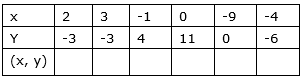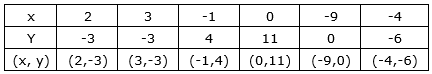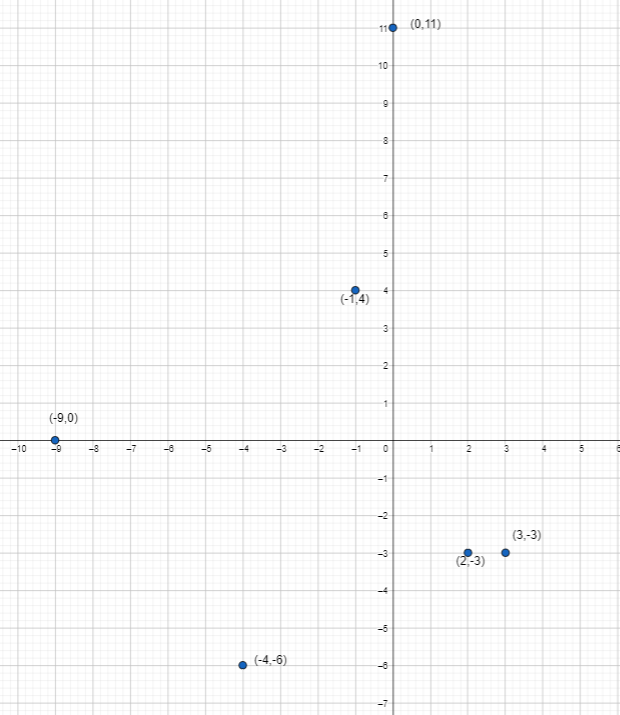Question 2.

Are the positions of (5, -8) and (-8, 5) is same? Justify your answer.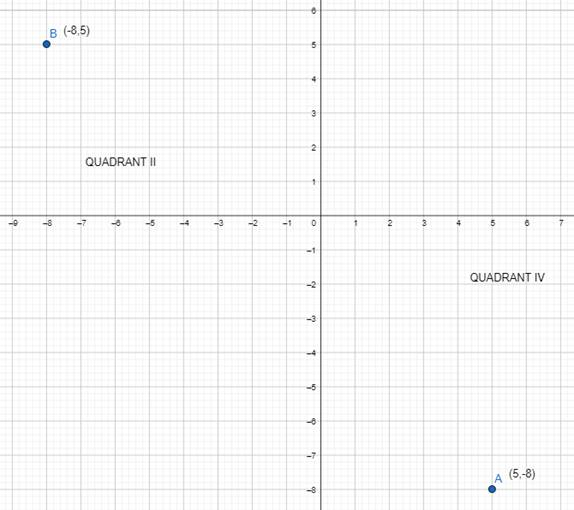No, positions of (5, -8) and (-8, 5) is not same because (5, -8) lies in IV quadrant and (-8, 5) lies in II quadrant.

Question 3.

What can you say about the position of the points (1, 2), (1, 3), (1, -4), (1, 0), and(1, 8). Locate on a graph sheet.

From the given points (1, 2), (1, 3), (1, -4), (1, 0), and(1, 8), we can say that all these points lie on a line parallel to Y-axis at a distance of 1 unit.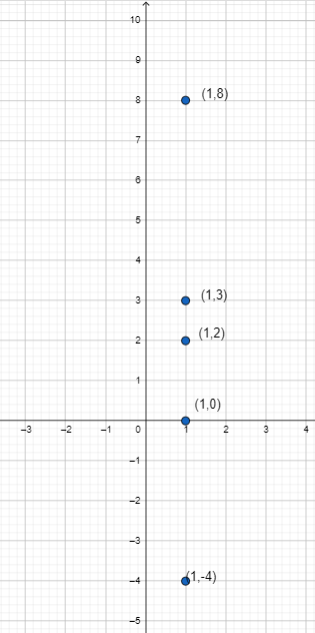Question 4.

What can you say about the position of the points (5, 4), (8, 4), (3, 4), (0, 4),(-4, 4), (-2, 4)? Locate the points on a graph sheet and justify your answer.

From the given points (5, 4), (8, 4), (3, 4), (0, 4),(-4, 4), (-2, 4), we can say that all these points lie on a line parallel to X-axis at a distance of 4 units.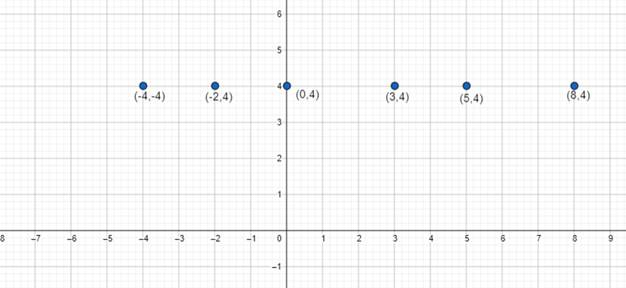Question 5.

Plot the points (0, 0),(0, 3), (3, 4), (4, 0) in graph sheet. Join the points with straight lines to make a rectangle. Find the area of the rectangle.

Plotting the points :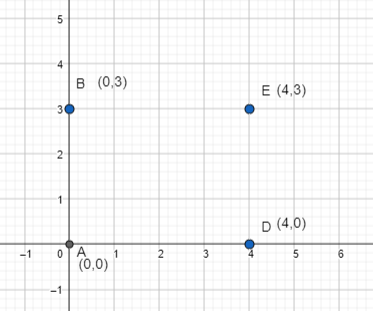Joining the lines to form a rectangle and finding its area :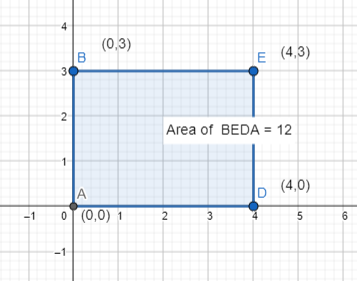Finding the area :

From the graph, we can see that,

L = AD = 4 units

B = ED = 3 units

Now, area of rectangle = L × B = 4 × 3 = 12 sq. unit.

∴ Area of rectangle BEDA = 12 sq. unit.

Question 6.

Plot the points (2, 3), (6, 3) and (4, 7) in a graph sheet. Join them to make it a triangle. Find the area of the triangle.

Plotting the points :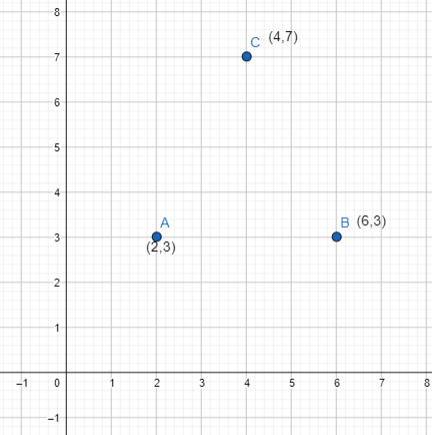Joining the points to form a triangle and finding its area :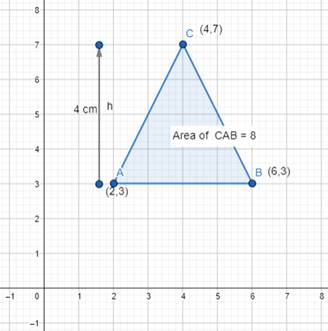Finding the area :

From the graph, we can see that,

H = 4 units

B = AB = 4 units

Area of the triangle =× B × H× 4 × 4 = 8 sq. unit.

∴ Area of triangle CAB = 8 sq. unit.

Question 7.

Plot at least six points in a graph sheet, each having the sum of its coordinates equal to 5. {Hint : ( -2, 7) (1, 4) .............}

The points whose sum of co-ordinates is equal to 5 are :

(2,3), (3,2), (0,5), (5,0), (1,4), (4,1).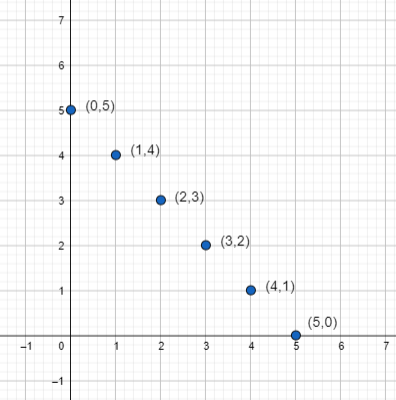Question 8.

Look at the graph. Write the coordinates of the points A, B, C, D, E, F, G, H, I, J, K, L,L, M, N, P, O and Q.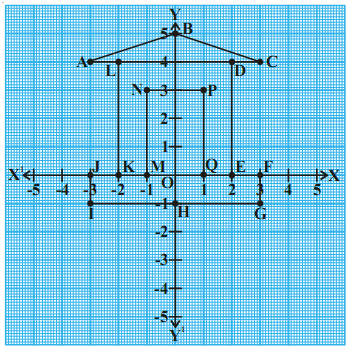From the graph, we can see that co-ordinates of the given points are :

Co-ordinates of point A are : (-3,4)

Co-ordinates of point B are : (0,5)

Co-ordinates of point C are : (3,4)

Co-ordinates of point D are : (2,4)

Co-ordinates of point E are : (2,0)

Co-ordinates of point F are : (3,0)

Co-ordinates of point G are : (3,-1)

Co-ordinates of point H are : (0,-1)

Co-ordinates of point I are : (-3,-1)

Co-ordinates of point J are : (-3,0)

Co-ordinates of point K are : (-2,0)

Co-ordinates of point L are : (-2,4)

Co-ordinates of point M are : (-1,0)

Co-ordinates of point N are : (-1,3)

Co-ordinates of point O are : (0,0)

Co-ordinates of point P are : (1,3)

Co-ordinates of point Q are : (1,0)

Question 9.

In a graph Sheet Plot each pair of points, join them by line segments

i. (2, 5), (4, 7)

ii. (-3, 5), (-1, 7)

iii. (-3, -4), (2, -4)

iv. (-3, -5), (2, -5)

v. (4, -2), (4, -3)

vi. (-2, 4), (-2, 3)

vii. (-2, 1), (-2, 0)

Now join the following pairs of points by straight line segments, in the same graph.

viii. (-3, 5), (-3, 4)

ix. (2, 5), (2, -4)

x. (2, -4), (4, -2)

xi. (2, -4), (4, -3)

xii. (4, -2), (4, 7)

xiii. (4, 7), (-1, 7)

xiv. (-3, 2), (2, 2)

Now you will get a surprise figure.

What is it?

Plotting the first set of points and forming their line segments :

i. (2, 5), (4, 7) → A,B

ii. (-3, 5), (-1, 7) → C,D

iii. (-3, -4), (2, -4) → E,F

iv. (-3, -5), (2, -5) → G,H

v. (4, -2), (4, -3) → I,J

vi. (-2, 4), (-2, 3) → K,L

vii. (-2, 1), (-2, 0) → M,N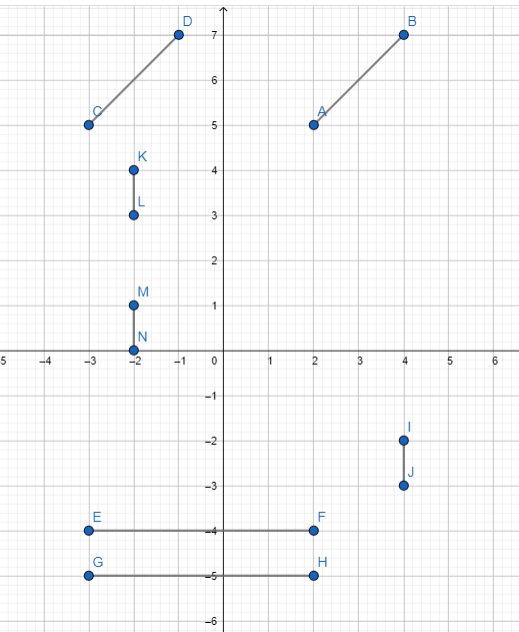Plotting the second set of points and forming their line segments :

viii. (-3, 5), (-3, 4) → O,P

ix. (2, 5), (2, -4) → Q,R

x. (2, -4), (4, -2) →S,T

xi. (2, -4), (4, -3) → U,V

xii. (4, -2), (4, 7) → W,X

xiii. (4, 7), (-1, 7) →Y,Z

xiv. (-3, 2), (2, 2) → A1,B1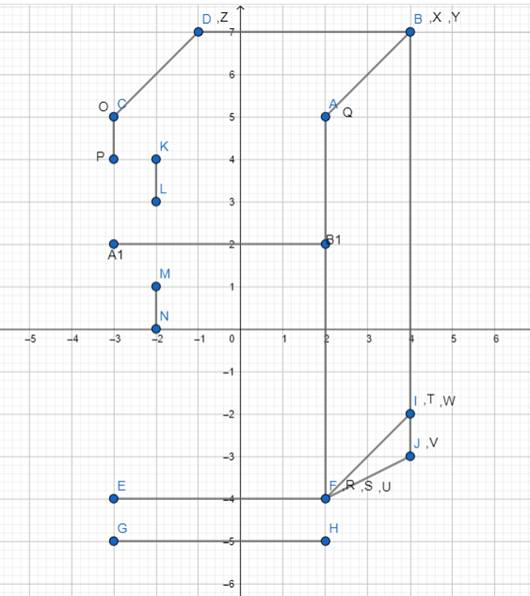## MATHS VIDEOS